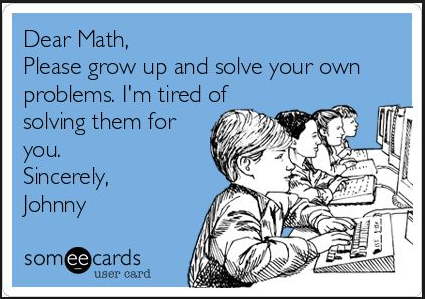Solving Systems of Equations (shorter version)
4 years ago
caityarmstrong
Save
Edit
Host a game
Live GameLive
Homework
Solo Practice
Practice10 QuestionsShow answers
• Question 1
300 seconds
Q. Solve for x and y
3x + 2y = 16
7x + y = 19
(-2,5)
(-2,-5)
(2,-5)
(2,5)
• Question 2
300 seconds
Q. Solve for x and y
y = 2x + 1
y = 4x - 1
(1,3)
(-1,-3)
(-1,3)
(3,1)
• Question 3
300 seconds
Q. The equations of two lines are:
2x-y=4 and y=-2x+8.
What is the value of x in the solution for this system?
x=8
x=3
x=11
x=5
• Question 4
300 seconds
Q. Christian had brochures printed for a new business venture. Christian originally ordered 4 boxes of black-and-white brochures and 3 boxes of color brochures, which cost a total of \$134. After those ran out, Christian spent \$120 on 3 boxes of black-and-white brochures and 3 boxes of color brochures. Which system represents this situation?
x+y=134
x+y=120
3x+3y=134
4x+3y=120
4x+3y=134
3x+3y=120
7xy=134
6xy=120
• Question 5
300 seconds
Q. Two brothers went shopping at a back-to-school sale where all shirts and shorts were the same price. The younger brother spent \$175 on 7 new shirts and 7 pairs of shorts. The older brother purchased 6 new shirts and 7 pairs of shorts and paid a total of \$165. How much did one shirt cost?
\$5
\$10
\$15
\$20
• Question 6
120 seconds
Q. If a system of equations has no solution, what does the graph look like?
intersecting lines
parallel lines
skew lines
intersecting lines
• Question 7
300 seconds
Q. What is the value of the y-coordinate of the solution to the system of equations
x-2y=1
x+4y=7
1
-1
3
4
• Question 8
60 secondsQ. What is the solution?
(1, -1)
(-1, 1)
(0, -2)
(2, 0)
• Question 9
300 seconds
Q. David is running a concession stand at a soccer game. He sells nachos and sodas. Nachos cost \$1.50 each and sodas cost \$0.50 each. At the end of the game, David made a total of \$78.50 and sold a total of 87 nachos and sodas combined. Which system of equations represents this situation?
1.5x+0.5y=78.5
x+y=87
1.5x+0.5y=78.5
1.5x+0.5y=87
x+y=78.5
1.5x+0.5y=87
x+y=78.5
x+y=87
• Question 10
60 secondsQ. What is the solution?